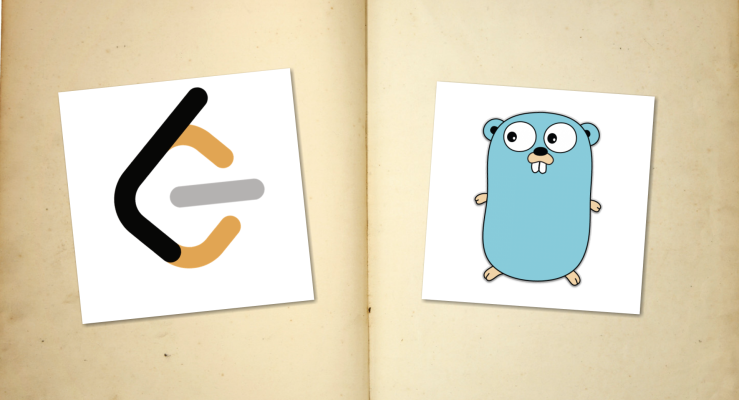# [Leetcode X Golang] Study Note 03

By: Axe 2019-10-01

## Code:

``````import "math"
func lengthOfLongestSubstring(s string) int {
window := make(map[byte]bool)
var l, i, j, r int
l = len(s)

for i < l && j < l {
if _, ok := window[s[j]]; ok {
delete(window, s[i])
i++
} else {
window[s[j]] = true
j++
r = int(math.Max(float64(r), float64(j - i)))
}
}
return r
}
``````

## Code Optimized:

``````import "math"
func lengthOfLongestSubstring(s string) int {
var charset int
i := 0
r := 0
for j, ch := range s {
i = int(math.Max(float64(charset[ch]), float64(i)))
r = int(math.Max(float64(r), float64(j - i + 1)))
charset[ch] = j + 1
}
return r
}
``````

## Notes:

• golang has no set type since no generics in go
• to delete key in golang, use `delete(map, key)`
• there is no max function for int, have to convert to float64 first
• when loop thru string, each char is byte type, and it's magically assignable to int array.